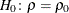Overview: CORR Procedure

The CORR procedure computes Pearson correlation coefficients, three nonparametric measures of association, polyserial correlation coefficients, and the probabilities associated with these statistics. The correlation statistics include the following:

• Pearson product-moment correlation

• Spearman rank-order correlation

• Kendall’s tau-b coefficient

• Hoeffding’s measure of dependence,• Pearson, Spearman, and Kendall partial correlation

• polychoric correlation

• polyserial correlation

Pearson product-moment correlation is a parametric measure of a linear relationship between two variables. For nonparametric measures of association, Spearman rank-order correlation uses the ranks of the data values and Kendall’s tau-b uses the number of concordances and discordances in paired observations. Hoeffding’s measure of dependence is another nonparametric measure of association that detects more general departures from independence. A partial correlation provides a measure of the correlation between two variables after controlling the effects of other variables.

Polyserial correlation measures the correlation between two continuous variables with a bivariate normal distribution, where only one variable is observed directly. Information about the unobserved variable is obtained through an observed ordinal variable that is derived from the unobserved variable by classifying its values into a finite set of discrete, ordered values.

A related type of correlation, polychoric correlation, measures the correlation between two unobserved variables with a bivariate normal distribution. Information about these variables is obtained through two corresponding observed ordinal variables that are derived from the unobserved variables by classifying their values into finite sets of discrete, ordered values.

When only one set of analysis variables is specified, the default correlation analysis includes descriptive statistics for each analysis variable and pairwise Pearson correlation statistics for these variables. You can also compute Cronbach’s coefficient alpha for estimating reliability.

When two sets of analysis variables are specified, the default correlation analysis includes descriptive statistics for each analysis variable and pairwise Pearson correlation statistics between the two sets of variables.

For a Pearson or Spearman correlation, the Fisher’stransformation can be used to derive its confidence limits and a-value under a specified null hypothesis. Either a one-sided or a two-sided alternative is used for these statistics.

When the relationship between two variables is nonlinear or when outliers are present, the correlation coefficient might incorrectly estimate the strength of the relationship. Plotting the data enables you to verify the linear relationship and to identify the potential outliers. If ODS Graphics is enabled, scatter plots and a scatter plot matrix can be created via the Output Delivery System (ODS). Confidence and prediction ellipses can also be added to the scatter plot. See the section Confidence and Prediction Ellipses for a detailed description of the ellipses.

You can save the correlation statistics in a SAS data set for use with other statistical and reporting procedures.